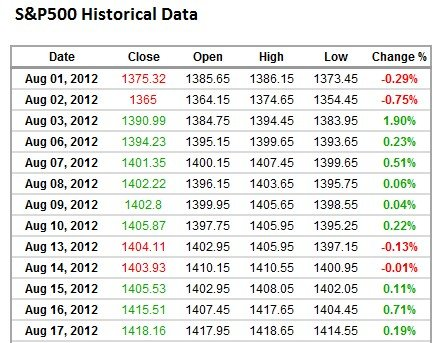# How to Calculate Volatility in Excel?

We know that the prices of different financial assets such as currencies and stocks are constantly fluctuating as traders buy and sell these assets. The variation in the prices over a period of time is called volatility. The volatility tells us about how turbulent the price is and is an indicator of the risk involved. A currency pair with high volatility involves high risk, but is also seen as an opportunity to make profits by the currency traders. If you trade in financial markets, then understanding volatility is important.

In this article, we will look at how the volatility can be calculated using excel. We will take the historical data for S&P 500 for the past three months and use the data to calculate the volatility.

Step 1: Get the Data

We have downloaded the price data for S&P500 in a spreadsheet. The data contains many things such as Close, Open, High, Low, and %change.

What we are interested in is the Closing price, and % change.Step 2: Calculate Return Series

Note that % Change is calculated using each day’s closing price and represents the return series that is the % change in price from one day to another. Even if the % change is not already given, it can be easly calculated. For example, the closing price on Aug 01, is 1375.32 and on Aug 02, it is 1365. %change on Aug 02 will be 1365/1375.32-1 = -0.75% as shown in the table above.

Our next step is to calculate the standard deviation of the daily returns. In excel the Standard Deviation is calculated using the =StdDev(). This formula takes the range of data as its input such as the % change data. The standard deviation can be calculated for any period such as 10-days, 30-days, or for the entire price.

Our standard deviation for the 3-month data is:

= StdDev(Data range for % Change)

= 0.78%

This standard deviation represents the volatility.

Calculate Annualized Volatility

Note that in the above calculation, we have used the daily data to calculate the standard deviation. This will be the 1-day volatility. We need to convert this into Annualized Volatility. Assuming that there are 252 trading days, the volatility can be annualized using the square root rule, as follows:

Annualized Volatility = 1-day volatility *Sqrt(252)

= 0.78%*Sqrt(252) = 12.38%

Note that if we had used weekly data instead of daily data, we will use Sqrt(52) as there are 52 weeks in a year.

Member-only